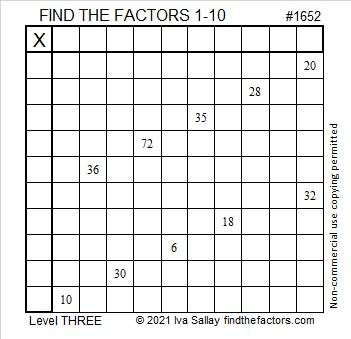# 1652 Start at the Top and Work Your Way Down to the Bottom

Contents

### Today’s Puzzle:

This is a level 3 puzzle so the clues are given in a logical order starting from the top of the puzzle. Begin by writing the factors of 20 and 32 in the appropriate cells. Then write the rest of the numbers so that both the first column and the top row have all the numbers from 1 to 10, and the written numbers are the factors of the given clues.### Factors of 1652:

• 1652 is a composite number.
• Prime factorization: 1652 = 2 × 2 × 7 × 59, which can be written 1652 = 2² × 7 × 59.
• 1652 has at least one exponent greater than 1 in its prime factorization so √1652 can be simplified. Taking the factor pair from the factor pair table below with the largest square number factor, we get √1652 = (√4)(√413) = 2√413.
• The exponents in the prime factorization are 2, 1, and 1. Adding one to each exponent and multiplying we get (2 + 1)(1 + 1)(1 + 1) = 3 × 2 × 2 = 12. Therefore 1652 has exactly 12 factors.
• The factors of 1652 are outlined with their factor pair partners in the graphic below.### More About the Number 1652:

1652 is the difference of two squares two different ways:
414² – 412² = 1652 and
66² – 52² = 1652.

This site uses Akismet to reduce spam. Learn how your comment data is processed.